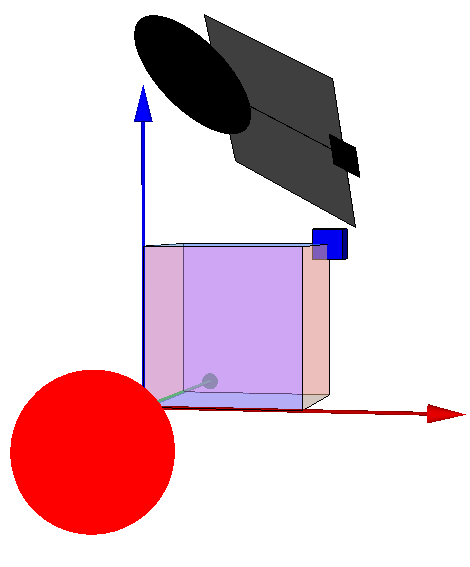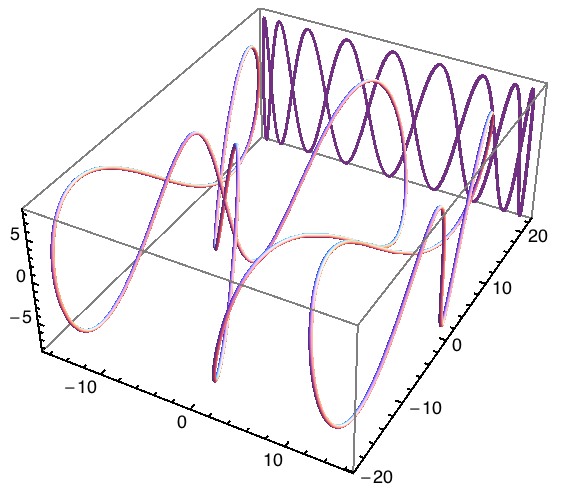# Shadows of 3D plots and objects

### Prof. Jens Nöckel, University of Oregon

This is the HTML version of a Mathematica 8 notebook. You can copy and paste the following into a notebook as literal plain text. The original code was posted on StackExchange.

On the Mathematica graphics main page, I point out the usefulness of the old Mathematica commands `Shadow` and `ShadowPlot3D`.

These commands project a 3D object onto one or several of the six faces of the bounding box. I've created my own projection function that generalizes this idea to arbitrarily tilted planar surfaces. The function has the ingenious name `planarShadow` and is listed below.

Strictly speaking, there is a big difference between the shadow of a 3D object and a projection. Shadows can be complete or partial, can be cast by the object on itself and on other surfaces, and depend on many other properties of the light source (such as its size, or whether there is diffuse lighting, ambient occlusion etc.). But in Mathematica graphics, it is unwise to try and be photorealisitc.

Therefore, the only difference between a shadow and a projection that I account for is the use of color: shadows are shades of gray, whereas projections should retain the object's surface colors. I am not simulating the blur effect that you find in many "fake shadows" such as the ones around Mac OS X window frames. Casting shadows on curved surfaces is also not something I would try in Mathematica. There are many 3D modeling programs that can do this sort of ray tracing much better. However, if you want to go further, a blur effect in 3D can in principle be created by using the method I describe on this page dealing with 3D text labels. For curved surfaces, one could texture each polygon of a surface according to its specific orientation.

```planarShadow[x_, direction_, normal_, darkShadow_:True] :=
Module[{d, n},
d = Normalize[direction];
n = Normalize[normal];
x /. Graphics3D[gr_, opts___] :>
GeometricTransformation[
gr /. {Glow[_] -> Glow[],
r_?(MemberQ[{RGBColor, Hue, CMYKColor, GrayLevel},
Composition[TranslationTransform[direction],
Quiet[
RotationTransform[{d, n}], {RotationMatrix::degen, RotationTransform::spln}
],
ScalingTransform[10^-3, d],
Quiet@Check[ScalingTransform[1./(n.d), n - (n.d) d],
Identity]]]}, opts]
]
```

The argument `x` is a 3D plot or graphics object. The second variable, `direction`, is parallel to the light rays and its length is equal to the offset between the object and its shadow. The third argument, `normal`, is the normal vector of the surface onto which the shadow is projected.

I also added an optional argument, `darkShadow`, that you can set to `False` if you want the projection to appear in color, instead of being darkened (that's the default). See the example at the bottom of this page for an application where a colored shadow may be more desirable.

### Examples

To illustrate this, I'll define a sample object (displayed below with its shadow):

```gg = Graphics3D[{{Opacity[.5], Cuboid[]}, {Blue,
Translate[Scale[Cuboid[], .2], {1, 1, 1}/2]}, , {Glow[Red], Red,
Translate[Scale[Sphere[], .5], -{1, 1, 1}/4]}}, Boxed -> False]
```

The sphere is made to glow, whereas the central cube has 50% opacity. I did this to illustrate how the shadow is affected by them. The glow will be ignored, but the opacity will affect the darkness of the shadow.

Now display it with some coordinate axis for orientation, assuming light going in the direction `{0,1,1}` and falling on a surface tilted into the space diagonal `{1,1,1}`:

```Show[gg,
planarShadow[gg, 2.1 {0, 1, 1}, {1, 1, 1}],
Graphics3D[{Map[{Apply[RGBColor, #], Arrow[Tube[{{0, 0, 0}, #}]]} &,
2 IdentityMatrix]}]
]
```The projection is stretched if the shadow surface isn't perpendicular to the rays. Of course there is the special case where the shadow surface is at a grazing angle to the light. I decided to handle this by not stretching the shadow.

Also observe that translucent regions create less dark shadows. And the whole thing is still a 3D object, not a bitmap.

Another example:

```Show[gg,
planarShadow[gg, -1.5 {0, 0, 1}, {0, 1, 1}],
Graphics3D[{Map[{Apply[RGBColor, #], Arrow[Tube[{{0, 0, 0}, #}]]} &,
2 IdentityMatrix]}]
]
```#### Using shadows in 3D plots

Now an example that shows how to use the above projection function in a 3D plot, similarly to the `ShadowPlot3D` command:

```With[{
a = Show[
ParametricPlot3D[{16 Sin[t/3], 15 Cos[t] + 7 Sin[2 t],
8 Cos[3 t]}, {t, 0, 8 \[Pi]}, PlotStyle -> Tube[.2],
AxesStyle -> Directive[Black, Thickness[.004]]],
TextStyle -> {FontFamily -> "Helvetica", FontSize -> 12},
DefaultBoxStyle -> {Gray, Thick}]
},
Show[a, planarShadow[a, {0, 22, 0}, {0, 1, 0}, False],
PlotRange -> All]]
```noeckel@uoregon.edu